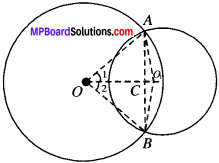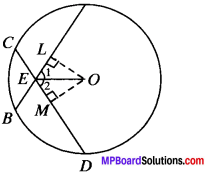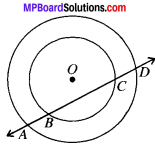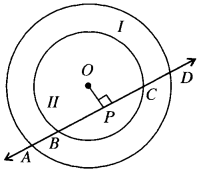# MP Board Class 9th Maths Solutions Chapter 10 Circles Ex 10.4

## MP Board Class 9th Maths Solutions Chapter 10 Circles Ex 10.4

Question 1.
Two circles of radii 5 cm and 3 cm intersect at two points and the distance between their centres is 4 cm. Find the length of the common chord.
Solution:
Given
Let O and O1 be the centre of bigger and smaller circle respectively
OA = OB = 5 cm
O1A – O1B = 3 cm
OO1 = 4 cmTo find: AB.
Construction:
Join OA, OB, O1A and O1B join AB also.
In ∆OAO1 and ∆OBO1
OA = OB (Radii of a circle)
O1A = O1B (Radii of a circle)
OO1 = OO1 (Common)
so ∆OAO1 = ∆OBO1 (By SSS)
and so ∠1 = ∠2 (By CPCT)
In ∆OCA and ∆OCB,
OA = OB (Radii of a circle)
∠1 = ∠2 (Proved)
OC = OC (Common)
∆OCA = ∆OCB (By SAS)
so AC = BC (By CPCT)
and ∠ACO = ∠BCO (By CPCT)
∠ACO + ∠BCO = 180° (LPA’s)
⇒ ∠ACO + ∠BCO = 180°
2∠ACO = 180°
∠ACO = 90°
ar (OAO1) = $$\frac{1}{2}$$ x OO1 x AC
= $$\frac{1}{2}$$ x 4 x AC = 2ACcm2 …..(i)
In ∆QAO1, a = 5 cm, bc = 4 cm, c = 3 cm
12
s = $$\frac{5+4+3}{2}$$ = $$\frac{12}{2}$$ = 6 cm
s – a = 6 – 5 = 1 cm
s – b = 6 – 4 = 2 cm
s – c = b – 3 = 3 cm
ar(OAO1) = $$\sqrt{s(s-a)(s -b)(s- c)}$$.
= $$\sqrt{6x1x2x3}$$
= 6 cm2 …..(ii)
From (i) and (ii), we get
2AC =6
AC = 3 cm
Now, AB = 2AC (∴ AC = BC)
= 2 x 3 = 6 cm.Method II. By Construction:
Geometrically, AB is the diameter of the circle of radius 3 cm as it passes through centre O1
AB = 2 x 3 = 6 cm.

Question 2.
If two equal chords of a circle intersect within the circle, prove that the segments of one chord are equal to corresponding segment of the other chord.
Solution:
Given
C (O, r) is a circle in which AB and CD are two equal chords which intersect at P.
To prove:
CP = BP and AP = DP.
Construction:
Draw OE and OF perpendiculars on AB and CD respectively. Join OP.
Proof:
In ∆OPF and ∆OPE,
OP = OP (Common)
OE = OF (∴ AB = CD)
∠F = ∠E (Each 90°)
∆OPF = ∆OPE (By RHS)
and so PE = PF …..(1) (By CPCT)
AB = CD (Given)$$\frac{1}{2}$$ AB = $$\frac{1}{2}$$ CD
BE = CF
and AE = DF …..(2)
Adding (1) and (2), we get,
PE + AE = PF + DF
∴ AP = DP
Subtracting (1) and (2) we get,
BE – PE = CF – PF
∴ BP = CP

Question 3.
If two equal chords of a circle intersect within the circle, prove that the line joining the point of intersection to the centre makes equal angles with the chords.
Solution:
Given
AB and CD are two equal chords of a circle which intersect at E.
To prove:
∠1 = ∠2
Construction:
Draw OL ⊥ AB and OM ⊥ CD. Join OE.Proof:
In ∆OLE and ∆OME,
OE = OE
OL = OM (∴ AB = CD)
∠L = ∠M (Each 90°)
∆OLE = ∆OME (By RHS)
and so∠1 = ∠2 (By CPCT)

Question 4.
If a line intersects two concentric circles (circles with the same centre) with centre O at A, B, C and D, prove that AB = CD. (see Fig. adjacent)Solution:
Given:
C (O, r) and C (O, r) are two concentric circles.
To prove: AB = CDProof:
AP = DP …(1)
In circle II, BC is the Chord and OP L BC.
∴ BP = CP …(2)
Subtracting (1) and (2), we get
AP – BP = DP – CP
AB = CDQuestion 5.
Three girls Reshma. Salma and Mandip are playing a game by standing on a circle of radius 5 m drawn in a park. Reshma throws a ball to Salma, Salma to Mandip, Mandip to Reshma. If the distance between Reshma and Salma and between Salma and Mandip is 6 m each, what is the distance between Reshma and Mandip?
Solution:
Given:
OR = OM = 5 m and SR = SM = 6 m.
To find: MR.Constrution:
Join OR, OM and OS. Draw ON ⊥ SR. In AORS and AOMS,
OS = OS (Common)
RS = MS (Given)
OR = OM (Given)
∆ORS = ∆OMS (By SSS)
and ∠1 = ∠2 (By CPCT)
SP = SP (Common)
SR = SM (Given)
∠1 = ∠2 (Proved)
∆SPR = ∆SPM (By SAS)
and so PR = PM (By CPCT)
and ∠3 = ∠4 (By CPCT)
∠3 + ∠4 = 180° (LPA’s)
2∠3 = 180°
∠3 = $$\frac{180^{\circ}}{2}$$ = 90°
ar (∆OSR) = $$\frac{1}{2}$$ x OS x PR …(i)
= $$\frac{1}{2}$$ x 5 x PR
ON ⊥ SR
RN = $$\frac{1}{2}$$ SR
(Perpendicular drawn from the centre of a circle to a chord bisects the chord)
= $$\frac{6}{2}$$ = 3m
In ∆ONR ON2 = $$\sqrt{O R^{2}-N R^{2}}$$ (Using Pythagoras Theorem)
= $$\sqrt{5^{2}-3^{2}}$$ = $$\sqrt{4^{2}}$$ = 4m
ar (∆OSR) = $$\frac{1}{2}$$ x SR x ON
= $$\frac{1}{2}$$ x 6 x $$\frac{1}{2}$$ x 4 = 12m2 …..(ii)
From (i) and (ii), we get
PR = $$\frac{2×12}{2}$$ = 4.8m
MR = 2 PR
= 2 x 4.8
= 9.6 mQuestion 6.
A circular park of radius 20 m is situated in a colony. Three boys Ankur, Syed and David are sitting at equal distance on its boundary each having a toy telephone in his hands to talk each other. Find the length of the string of each phone.
Solution:
Given: OS = OA = 20 m and AS = SD = AD
To find: AS, SD and AD.
Construction:
Draw AE ⊥ SD. Join OS.
Let AS = SD = AD = 2x (say)In equilateral ∠ASD, AE ⊥ SD
⇒ E is the mid-point of SD
SE = $$\frac{2x}{2}$$ = x
In ∆AES, AS2 = AE2 + SE2
(2x)2 = AE2 + x2
4x2 – x2 = AE2
AE = $$\sqrt{3x^{2}}$$ = $$\sqrt{3}$$
OE = AE – AO
= ($$\sqrt{3}$$ – 20)m
In ∆OES, OS2 = OE2 + SE2
(20)2 = [($$\sqrt{3}$$x) 20]2 + x2
400 = ($$\sqrt{3}$$x)2 – 2 x $$\sqrt{3}$$x × 20 + (20)2 + x2
= 3x2 + 400 – 40$$\sqrt{3}$$x + x2
400 – 400 = 4x2 – 40$$\sqrt{3}$$x
0 = 4x2 – 40$$\sqrt{3}$$x
40$$\sqrt{3}$$x = 4x2
40$$\frac { 40\sqrt { 3 } }{ 4 }$$ = x
x = 10$$\sqrt{3}$$m .
2x = 2 x 10$$\sqrt{3}$$ = 20$$\sqrt{3}$$m
AS = SD = AD = 20$$\sqrt{3}$$m.